In the following we introduce some basic concepts which will constitute ur main conceptual framework for the job to learn a task. The main overview is given in the figure 11.1, a more spcialized view will be shown in figure 11.2.Presupposing the concepts 'Learning System (LS)' and 'Task (T)' as part of an environment we can illustrate all important concepts.

1. The set of observable stimulus-response pairs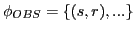can represent a random set, a partially deterministic-static or a partially deterministic-dynamic set. 'deterministic' means that a '(s,r)-pair' occurs as some 'constant. If the set of all constant (s,r)-pairs is 'static', then the system doesn't act randomly but in a static, fixed manner. In tis case the system can not react to changes in an environment. in the 'dynamic' case the system can increase or decrease the set of (s,r)-pairs which are constant. This allows the system to respond to changes in the environment.
2. A random empirical behavior functioncorresponds to a theoretical system function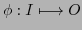which has no specialized internal states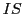.
3. A deterministic static empirical behavior functioncorresponds to a theoretical system functionwhich has fixed specialized internal stateswhich determine certain responses, which are static.
4. A deterministic dynamic empirical behavior functioncorresponds to a theoretical system function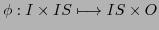which has specialized internal stateswhich determine certain responses, which are dynamic growing depending from the different tasks.

These are the basic concepts. These do not include the interaction with a defined task. If one has an explicitly defined task as a kind of a benchmark including initial states, intermediate states as well as goal states with at least one possible solution path, then one can introduce additional concepts.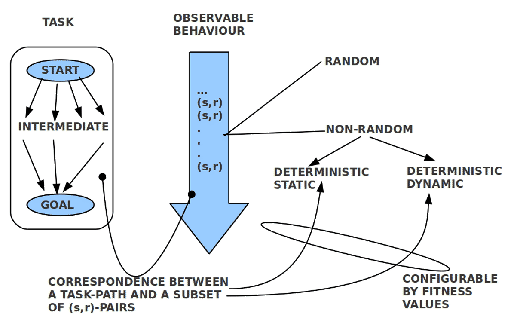1. One can distinguish between those deterministic (s,r)-pairs in a static or dynamic set of (s,r)-pairs which show no correlation with a solution path and those which do. Even more one can quantify the agreement between a solution path and the set of (s,r)-pairs with 100% if all steps in a solution path have a corresponding (s,r)-pair in the observable behavior.
2. While a set of possible (s,r)-pairs supported by a behavior function can be in agreement with a solution path or not it depends from some kind of evaluation whether such a set of (s,r)-pairs can be 'tailored' for a solution path or not. If such an evaluation is available as fitness value then a system can exploit these values to 'configure' its (s,r)-pairs in an appropriate 'order'. Thus we have two different meanings of 'dynamic': (a) being able to arrange a given set of (s,r)-pairs in a way which is in agreement with the 'fitness values' (in a 'fitness conformant way') or (b) being able to change the set of (s,r)-pairs .
3. The concept of learning will presuppose at least a configurability according to a fitness value. Additionally it can include the changeability of the set of (s,r)-pairs.
4. The general case of configurability according to a fitness value implies a set of available fitness values which reflect a minimal order to allow an appropriate arrangement of the (s,r)-pairs.
5. The change-case implies that for new stimuli new responses have to be selected. This presupposes a search space from which possible responses can be selected and as well a fitness space from which the system can infer those evaluations which allow learning.
6. Without any kind of preferences ('values') there can be no learning. Fitness values can be generated from the outside ('supervised') and from the inside('unsupervised').
7. Furthermore must a LS be able to perceive a change of a state as a stimulus.
8. For a LS is must be possible to associate a perceptionwith a fitness value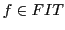having at least two different values.
9. A LS must be able to store a perception with the fitness value as well as to remember stored items from the past as well as to select from remembered items a possible best option for an actual possible action.
10. A LS must be able to cause a response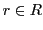which is able to trigger the change of a given state.

REMARK: During the past discussions it became clear, that one has to draw a clear distinction between two basic cases: (1) the fitness value in the context of a genetic population and (2) the fitness value in the context of the interactions of a phenotype with it's environment. While the fitness values in the case of populations of genes are realized by selecting those which survive and those which die, we have in the case of phenotypes the interactions of one phenotype with its environment and a fitness value in this framework signals some event which can change actual system parameters.Thus finding 'food' can be associated with some fitness value which will increase the system parameter 'energy'. This means that the system internally can use the system parameter 'energy' as a measure for a special fitness value. For to see how this works we have to look for more details during the upcoming experiments.

If we assume the system as a learning system then this implies that the system - in the worst case - has no 'knowledge' about the path from the start state to the goal state. To 'built up' such a knowledge the learning system has to search around to encounter those states which are necessary. While searching the learning system has to 'remember' all the encountered states and their possible relationships to a goal state. While in the search mode the system can produce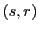-pairs by chance - having anproducing an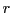- or by memory. If the set of stored-pairs increases by time - depending from the activity - the probability increases that the system can remember more and more intermediate states to find a path from a start state to a goal state. We will say that the set of-pairs constitutes the knowledgeof the system, which is a part of the system function. This means in connection with the formulawe have to assume that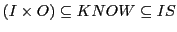andand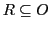.

Subsections
Gerd Doeben-Henisch 2013-01-14